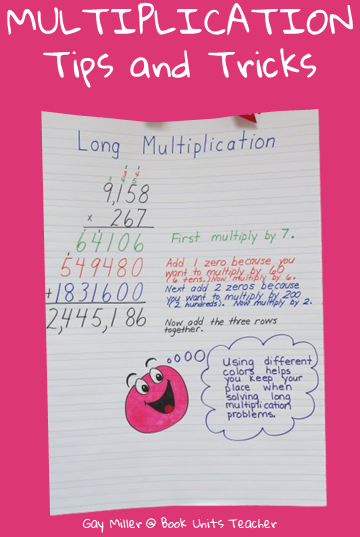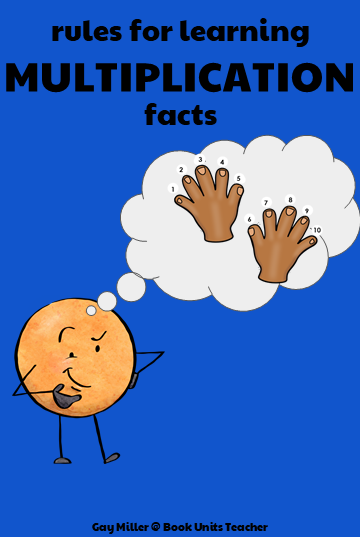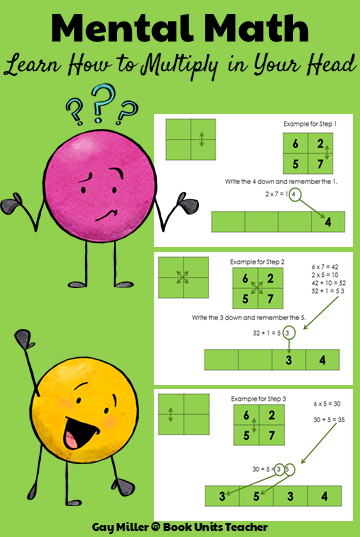# Multiplication Facts Tips and Tricks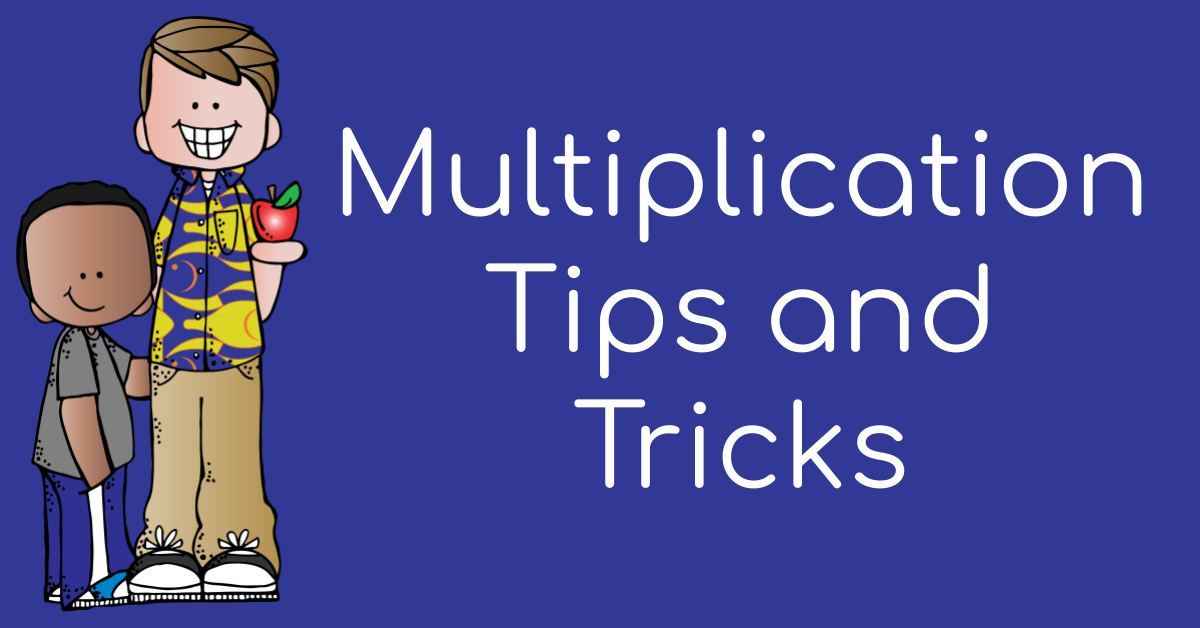Some students seem as if they learn multiplication facts overnight. Others struggle for months and months. Flashcards, using Brain Gym exercises while repeating facts, and catchy songs work with a few students. One method that works for many students is learning rules. Here is the list I have tried with success. Below is an instruction video that contains even more tricks or rules.

### Rules for Learning Multiplication Facts

#### Rule #1 – Any number times 1 equals the other number.

5 x 1 = 5           1 x 7 = 7

#### Rule #2 – Any number times 10 equals the other number plus a 0.

50 x 1 = 50           1 x 70 = 70

#### Rule #3 – Any number times 11 equals the other number doubled.

5 x 11 =55           11 x 7 = 77

#### Rule #4 – Skip count when multiplying by 2’s. (2, 4, 6, 8, 10, 12, 14, 16, 18)

5 x 2 = 10           2 x 7 = 14

#### Rule #5 – You can remember the 4’s by doubling twice.

5 x 4 = double 5 =10 so 10 +10=20           2 x 7 = double 7=14 so 14+14=28

#### Rule #6 – When you multiply 6 by an even number, they both end in the same digit.

2 x 6=12­        4 x 6=24        6 x 6=36        8 x 6=48

#### Rule #8 – Skip count when multiplying by 5’s. (5, 10, 15, 20, 25, 30, 35, 40, 45)

5 x 5 = 25           5 x 7 = 35

#### Rule #9 – Use your fingers. Bend down the finger you are multiplying by 9.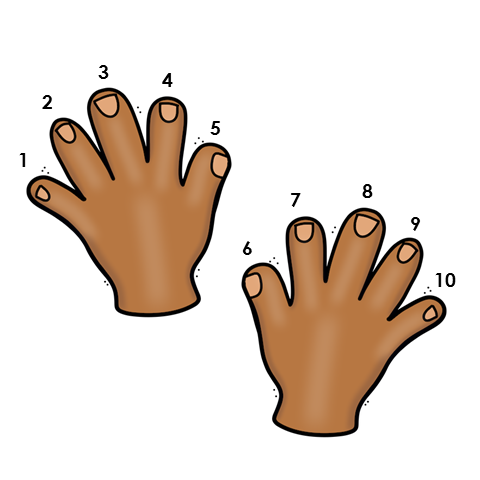For 5 x 9, bend down finger #5. You have 4 fingers on the left side of the bent finger and 5 fingers on the right side of the bent finger. The answer is 45.

For 9 x 7, bend down finger 7. You have 6 fingers on the left side of the bent finger and 3 fingers on the right side of the bent finger. The answer is 63.

Learn some more tips for memorizing the multiplication facts with this great instructional video.

### A Fun Way for Students Impress Friends ans Family

Once students have learned the multiplication facts 1-9, this trick is a great way  to “show off.”This multiplication trick only works with a very specific type of problem, but you can really impress your family and friends by solving this type of multiplication problem in record time if you are the only one that knows the ‘trick.’

The trick works when you multiply a 2 digit number by a 2 digit number if:

• the two digits in the tens place add up to 10
• the 2 digits in the ones place repeat

Example Problems

23 x 83
2 + 8 = 10

Both numbers in the ones place are 3’s.

91 x 11
9 + 1 = 10

The number 1 is in the ones place.

75 x 35
7 + 3 = 10

The ones place has the number 5.

64 x 44
6 + 4 = 10

Both numbers in the ones place are 4’s.

Here is how to solve the problem.

Step #1 -Multiple the numbers in the ones place and write down your answer in the ones and tens place.

thousands

hundreds

tens

ones

For 23 x 83, multiply 3 times three. The answer is 9. Write nine in the ones place and place a zero in the tens place.

thousands

hundreds

0

9

Step #2 – Multiply the two numbers in the tens place and then add one of the numbers in the ones place to that total.

For 23 x 83, multiply 2 x 8 and then add 3.

2 x 8 = 16 + 3 = 19 – Place this number in the thousands and hundreds place.

1

9

0

9

The end result is 23 x 83 = 1909.

Here is a list of problems that follow this pattern. Give them a try.

19 x 99 =

35 x 75 =

67 x 47 =

60 x 40 =

81 x 21 =

28 x 88 =

56 x 56 =

39 x 79 =

22 x 82 =

75 x 35 =

57 x 57 =

74 x 34 =

43 x 63 =

94 x 14 =

86 x 26 =

48 x 68 =

#### Another Strategy for Multiplying Two-Digit by Multiplying Two Digit Numbers

This method has three steps and is not quite as easy to do in your head as the previous method. It is not as limited as the first trick and you can use it with any 2-digit by 2-digit multiplication problem. Think of this process as a method for being able to do mental math. The strategy follows traditional multiplication steps.

##### Step #1

Multiply the 2 numbers in the ones places. Place the answer you get in the ones place in the ones place column. You must remember the number you get in the tens place.

#####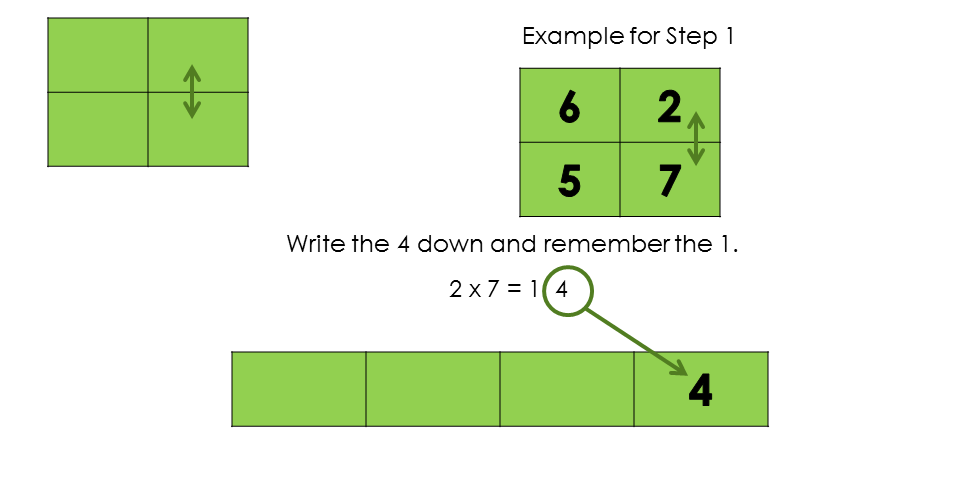Step #2

Multiply the numbers at opposite corners and add them together. Then add the number you remembered from Step #1.  Just like in Step #2, write the number you get in the ones place in the next box (tens place) and remember the other number.

#####Step #3

Multiply the two numbers in the left column and add the number you remembered from Step #3.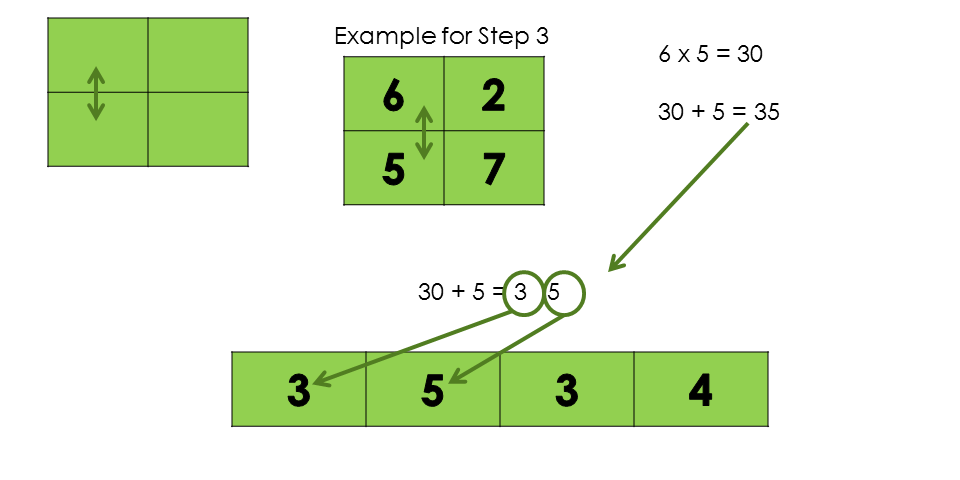### Get these tips and tricks in printable format.